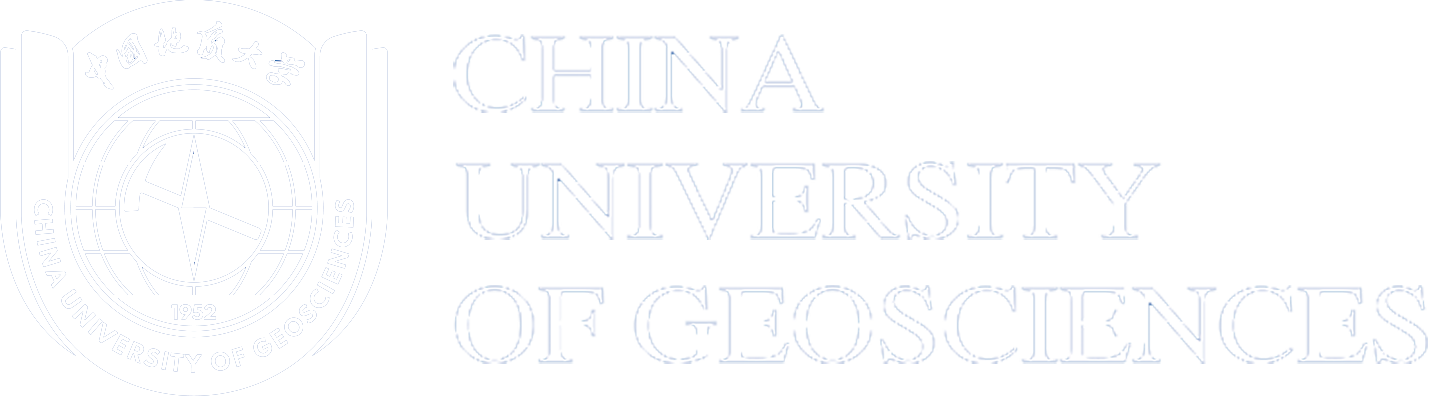#CUGB

1. Major Introduction

Mathematics studies quantity, spatial model, structure and change, a discipline to research the quantitative relations and spatial forms of the real world by using logic forms. The discipline of Mathematics and Applied Mathematics is to clarify the concept of mathematical science and the regularity of the phenomenon, a basic subject to make a study of mathematical theory in natural sciences, social sciences and engineering, aiming to train students’ knowledge of basic mathematic theories and practices and their ability to solve practical problems with mathematics and computers. This program lays emphasis on basic training and a wide range of applications, widens scope for training and takes computing and information science for the major professional development direction, fully demonstrating the basic and instrumental quality of mathematics.

Undergraduate program in Mathematics and Applied Mathematics cultivates professionals with basic theories and practice in mathematical sciences, ability to apply knowledge of mathematics and computers to solve practical problems, and with initial trainings of scientific research. Graduates are qualified for research and teaching in sectors like science and technology, education, economy and finance, for work of practical applications, development research and administration in departments of production, operation and administration, or for a higher academic degree.

Undergraduates of this program study basic theories and practice in mathematics and applied mathematics, receive basic training in mathematical model, computers and mathematical software, and are well educated both in mathematics theories and applications. Undergraduates have good scientific literacy and strong innovation consciousness, and abilities of scientific research, teaching, resolving practical problems, developing software and updating knowledge.

(1)  have a solid mathematical foundation, rigorous scientific thinking training, preliminary grasp of mathematical science thinking method.

(2)  develop ability to solve practical problems with mathematical modeling using mathematical knowledge.

(3)  acquaint with the history of scientific developments and future applications of some new development in modern mathematics.

(4)  familiarize with the use of computers (including the common language, tools software and mathematical software), and with simple program writing.

(5)  have a good proficiency of language, know how to search materials and literature with the help of modern information technology, and know how to conduct scientific research.

4. Length of Schooling and Degree

The length of schooling is four years of full-time study. Students will be awarded the Bachelor Degree of Science when they have completed the required minimum credits and have met all other requirements.

5. Core Courses

Specialized Core Courses: Ordinary Differential Equations, Mathematics Modeling, Mathematical Statistics, Numerical Analysis B, Equations of Mathematical Physics A, Numerical Solution of Partial Differential Equations, Combinatorial Mathematics, Financial Mathematics, Specialty English for Mathematics.

Practice Courses: Physics Experiments, Fundamentals and Applications of MATLAB, Mathematics Modeling Experiment, Statistical Analysis Software, Graduation Design (Thesis).